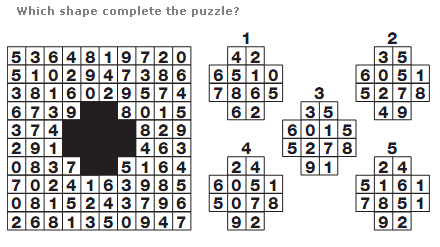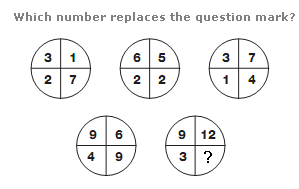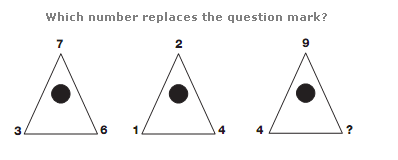# Puzzles - Number puzzles

Exercise : Number puzzles
58.:
4
Explanation
:
Each row of the grid contains each of the 10 digits, 0 to 9, written in a random order.

59.:
6
Explanation
:
Numbers in the segments of the lower left circle equal the sum of the numbers in the upper left and central circles. Numbers in the segments of the lower right circle equal the sum of the numbers in corresponding segments of the upper right and central circles.

60.